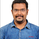Dilihat 2459
Uses two sets of MACD plus an RSI to either long or short. All three indicators trigger buy/sell as one (ie it's not 'IF MACD1 OR MACD2 OR RSI > 1 = buy", its more like "IF 1 AND 2 AND RSI=buy", all 3 match required for trigger)

The MACD inputs should be tweaked depending on timeframe and what you are trading. If you are doing 1, 3, 5 min or real frequent trading then 21/44/20 and 32/66/29 or other high value MACDs should be considered. If you are doing longer intervals like 2, 3, 4hr then consider 9/19/9 and 21/44/20 for MACDs (experiment! I picked these example #s randomly).
Ideal usage for the MACD sets is to have MACD2 inputs at around 1.5x, 2x, or 3x MACD1's inputs.

Other settings to consider: try having fastlength1=macdlength1 and then (fastlength2 = macdlength2 - 2). Like 10/26/10 and 23/48/20. This seems to increase net profit since it is more likely to trigger before major price moves, but may decrease profitable trade %. Conversely, consider FL1=MCDL1 and FL2 = MCDL2 + (FL2 * 0.5). Example: 10/26/10 and 22/48/30 this can increase profitable trade %, though may cost some net profit.

Feel free to message me with suggestions or questions.
```//@version=2
strategy("MACDbl RSI", overlay=true)

fastLength = input(10)
slowlength = input(22)
MACDLength = input(9)

MACD = ema(close, fastLength) - ema(close, slowlength)
aMACD = sma(MACD, MACDLength)
delta = MACD - aMACD

fastLength2 = input(21)
slowlength2 = input(45)
MACDLength2 = input(20)

MACD2 = ema(open, fastLength2) - ema(open, slowlength2)
aMACD2 = sma(MACD2, MACDLength2)
delta2 = MACD2 - aMACD2

Length = input(14, minval=1)
Oversold = input(20, minval=1)
Overbought = input(70, minval=1)
xRSI = rsi(open, Length)

if (delta > 0) and (year>2015) and (delta2 > 0) and (xRSI < Overbought)

if (delta < 0) and (year>2015) and (delta2 < 0) and (xRSI > Oversold)
strategy.entry("sell", strategy.short, comment="sell")

//plot(strategy.equity, title="equity", color=red, linewidth=2, style=areabr)```

## KomentarHi Ryan

I am newbie, how i can execute this script and get this trigger?
JawabKalghamdi12
from B<E ? is that an abbreviation of what the error message showed up or what it said verbatim? I couldn't get it to reproduce on my end. Perhaps it was the symbol you were looking up it somehow didn't like. If you give me the specifics of what symbol and time interval you were using I'll see if I can help.
Jawab